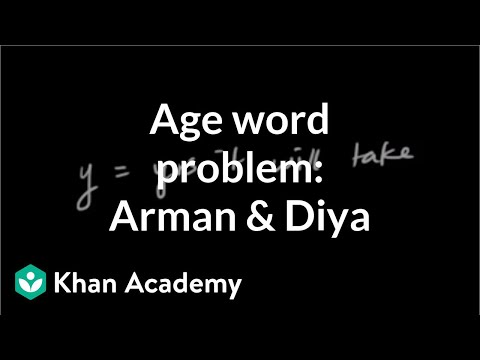Video

# Systems of equations with elimination: Sum/difference of numbers (Full video)

Description: Sal demonstrates solving a word problem by writing a system of equations and solving using the elimination method. And they tell us the sum of two numbers is 70. Let's construct an equation from this first sentence. Let's call those numbers x and y.

### Other videos you might be interested in### Solving Systems of Equations by Substitution (Full video)

#### Student Achievement Partners